# Representing 2D Transformations as Matrices

John E. Howland
Department of Computer Science
Trinity University
One Trinity Place
San Antonio, Texas 78212-7200
Voice: (210) 999-7364
Fax: (210) 999-7477
E-mail: jhowland@Trinity.Edu
Web: http://www.cs.trinity.edu/~jhowland/

June 28, 2004

### Abstract:

2D graphics transformations are represented as matrices. J programs for manipulating transformations such as scaling, rotation and translation are given. Efficiency of matrix representation of transformations is discussed.

Subject Areas: 2D Graphics Transformations.
Keywords: Modeling, J Programming Language, 2D Graphics Transformations.

# 1 Introduction

In these notes, we consider the problem of representing 2D graphics images which may be drawn as a sequence of connected line segments. Such images may be represented as a matrix of 2D points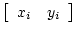. In the following pages we use the J [Hui 2001] programming notation to describe the various transformations.

For example:

   [ square =: 5 2 $0 0 10 0 10 10 0 10 0 0 0 0 10 0 10 10 0 10 0 0  represents the square shown in Figure 1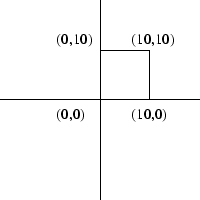The idea behind this representation is that the first point represents the starting point of the first line segment drawn while the second point represents the end of the first line segment and the starting point of the second line segment. The drawing of line segments continues in similar fashion until all line segments have been drawn. A matrix having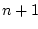points describes a figure consisting of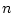line segments. It is sometimes useful to think of each pair of consecutive points in this matrix representation,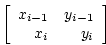as as a vector so that the square shown in Figure 1 is the result of drawing the vectors shown in Figure 2.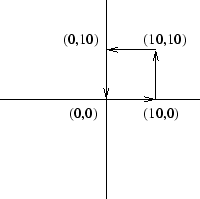# 2 Rotation Suppose we wish to rotate a figure around the origin of our 2D coordinate system. Figure 3 shows the point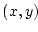being rotated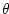degrees (by convention, counter clock-wise direction is positive) about the origin.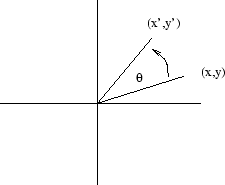The equations for changes in the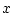and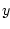coordinates are: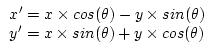(1) If we consider the coordinates of the pointas a one row two column matrix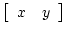and the matrix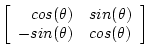then, given the J definition for matrix product, mp =: +/ . *, we can write Equations (1) as the matrix equation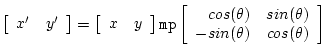(2) We can define a J monad, rotate, which produces the rotation matrix. This monad is applied to an angle, expressed in degrees. Positive angles are measured in a counter-clockwise direction by convention.  rotate =: monad def '2 2$ 1 1 _1 1 * 2 1 1 2 o. (o. y.) % 180'
rotate 90
0 1
_1 0
rotate 360
1 _2.44921e_16
2.44921e_16            1


We can rotate the square of Figure 1 by:

   square mp rotate 90
0  0
0 10
_10 10
_10  0
0  0


producing the rectangle shown in Figure 4.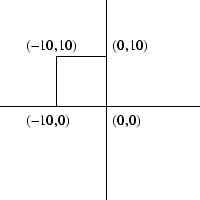# 3 Scaling

Next we consider the problem of scaling (changing the size of) a 2D line drawing. Size changes are always made from the origin of the coordinate system. The equations for changes in theandcoordinates are: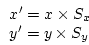(3)

As before, we consider the coordinates of the pointas a one row two column matrixand the matrix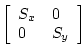then, we can write Equations (3) as the matrix equation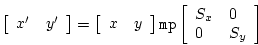(4)

We next define a J monad, scale, which produces the scale matrix. This monad is applied to a list of two scale factors forandrespectively.

   scale =: monad def '2 2 $(0 { y.),0,0,(1 { y.)' scale 2 3 2 0 0 3  We can now scale the square of Figure 1 by:  square mp scale 2 3 0 0 20 0 20 30 0 30 0 0  producing the square shown in Figure 5.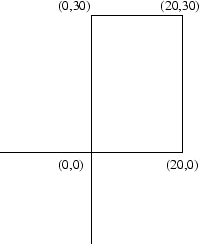# 4 Translation The third 2D graphics transformation we consider is that of translating a 2D line drawing by an amount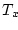along theaxis and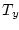along theaxis. The translation equations may be written as: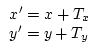(5) We wish to write the Equations 5 as a single matrix equation. This requires that we find a 2 by 2 matrix,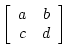such that. From this it is clear that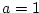and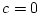, but there is no way to obtain theterm required in the first equation of Equations 5. Similarly we must have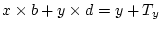. Therefore,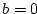and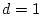, and there is no way to obtain theterm required in the second equation of Equations 5. ## 4.1 Homogenous Coordinates From the above argument we now see the impossibility of representing a translation transformation as a 2 by 2 matrix. What is required at this point is to change the setting (2D coordinate space) in which we phrased our original problem. In geometry, when one encounters difficulty when trying to solve a problem inspace, it is customary to attempt to re-phrase and solve the problem inspace. In our case this means that we should look at our 2D problem in 3 dimensional space. But how can we do this? Consider that, given a pointin 2 space, we map that point to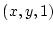. That is, we inject each point in the 2D plane into the corresponding point in 3 space in the plane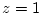. If we are able to solve our problem in this plane and find that the solution lies in the plane, then we may project this solution back to 2 space by mapping each pointto. To summarize, we inject the 2D plane into 3 space by the mapping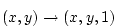(6) Then we solve our problem, ensuring that our solution lies in the plane. Our final answer is obtained by the projection of the planeon 2 space by the mapping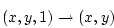(7) This process is referred to as using homogeneous coordinates. In the context of our problem (finding matrix representations of rotation, scaling and translation transformations) we must inject our 2D line drawings into the plane. In J we do this by using stitch, ,..  square ,. 1 0 0 1 10 0 1 10 10 1 0 10 1 0 0 1  We now must rewrite the Equations 5 as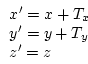(8) Consider the 3 by 3 matrix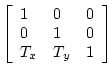We now see that the Equations 8 may be written as the matrix equation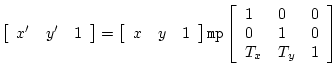(9) We define the J monad translate, which is applied to a list of two translate values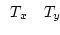.  translate =: monad def '3 3$ 1 0 0  0 1 0  , y. , 1'
translate 10 _10
1   0 0
0   1 0
10 _10 1


We translate the square of Figure 1 by

   (square ,. 1) mp translate 10 _10
10 _10 1
20 _10 1
20   0 1
10   0 1
10 _10 1


## 4.2 Efficiency of Transformations

Notice that the translate matrix (having a last column 0 0 1) always produces a result which lies in the plane. We can perform the translation operation and project the result back on the 2D plane (saving computation time by not doing unnecessary multiplications and additions) by

   (square ,. 1) mp 3 2 {. translate 10 _10
10 _10
20 _10
20   0
10   0
10 _10


producing the translated square shown in Figure 6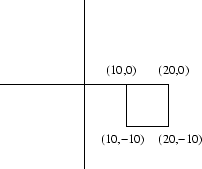# 5 Scaling and Rotation Using Homogeneous Coordinates

We want to be able to combine sequences of rotations, scaling and translations together as a single 2D graphics transformation. We accomplish this by simply multiplying the matrix representations of each transformation using matrix multiplication. However, to do this, we must go back and rewrite the Equations 1 and 3 as the following:(10)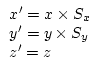(11)

Similarly we rewrite the matrix Equations 2 and 4 as: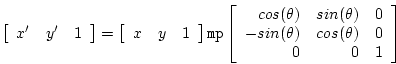(12)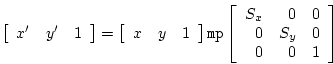(13)

We extend our earlier J definitions of rotate and scale to the homogenous coordinate system.

   rotate =: monad def '((2 2 $1 1 _1 1 * 2 1 1 2 o. (o. y.) % 180),.0),0 0 1' rotate 180 _1 0 0 0 _1 0 0 0 1 (square ,. 1) mp 3 2 {. rotate 180 0 0 _10 0 _10 _10 0 _10 0 0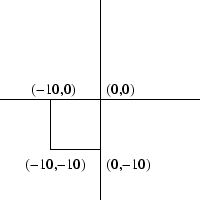scale =: monad def '3 3$ (0 { y.), 0 0 0 , (1 { y.), 0 0 0 1'
scale 2 3
2 0 0
0 3 0
0 0 1
(square ,. 1) mp 3 2 {. scale 2 3
0  0
20  0
20 30
0 30
0  0


Figure 5 shows the resulting scaled square.

# 6 Combining Transformations

We can now combine together two transformations to form a single graphics operation. For example, suppose we wish to first rotate an object 90 degrees and then scale the object by 2 along theaxis.

The rotation would be expressed as:

    [r =: rotate 90
0 1 0
_1 0 0
0 0 1


Then the scaling operation would be expressed as:

   [s =: scale 2 1
2 0 0
0 1 0
0 0 1


Applying these operations to the square, we have:

   (((square ,. 1) mp 3 2 {. r) ,. 1) mp 3 2 {. s
0  0
0 10
_20 10
_20  0
0  0


## 6.1 Efficiency of Operations

However, notice that

   (square ,. 1) mp 3 2 {. r mp s
0  0
0 10
_20 10
_20  0
0  0


produces the same result using far fewer multiplications and additions. Figure 8 shows the rotated and scaled square.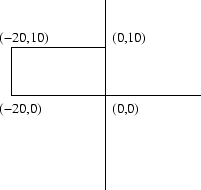We are allowed to perform the matrix multiplications of r and s before multiplying by square ,. 1 because matrix multiplication is associative.

Be careful! Matrix multiplication is not commumative.

   r mp s
0 1 0
_2 0 0
0 0 1
s mp r
0 2 0
_1 0 0
0 0 1


This means we must be careful about the order of application of graphics transformations.

One might be concerned about whether or not multiplying rotation, scaling and/or translation matrices produces a transformation which leaves our 2D lines in the plane. We can answer this question by observing that each of these matrices has a last column of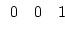. Hence, when multiplying any two of these matrices, the product matrix has a last column of.

# 7 Rotating an Object About a Point

As a final example, suppose we wish to rotate the square of Figure 1 90 degrees about its upper right corner. We must first translate the point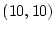to the origin. This is the matrix

   translate _10 _10
1   0 0
0   1 0
_10 _10 1


Then we must rotate 90 degrees

   rotate 90
0 1 0
_1 0 0
0 0 1


Finally, we translate the square back with the matrix

   translate 10 10
1  0 0
0  1 0
10 10 1


Putting this all together we have:

   [xform =: (translate _10 _10) mp (rotate 90) mp translate 10 10
0 1 0
_1 0 0
20 0 1
(square ,. 1) mp 3 2 {. xform
20  0
20 10
10 10
10  0
20  0


which is shown in Figure 9.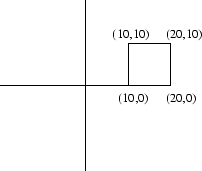## Bibliography

Hui 2001
Hui, Roger K. W., Iverson, Kenneth E., J Dictionary, J Software, Toronto, Canada, May 2001.

2005-01-18